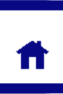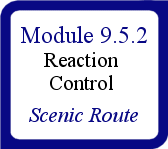# Equilibria continued...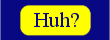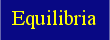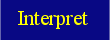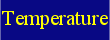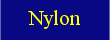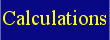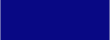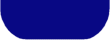Students learn to interpret the equilibrium constant expression (no units required) from the chemical equations of equilibrium reactions.

Part 2: Just to further illustrate these points and introduce the equilibrium constant, here are some more examples.

There exists an equilibrium reaction for the production of ammonia from nitrogen and hydrogen gas: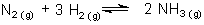Le Châtelier's Principle holds here as well. If we were to add ammonia to this system once it was already at equilibrium, the system would move to produce more of the reactants.

Equilibrium Constant:
Just to revise where the expression for the equilibrium constant, K, comes from, let's consider this general equation for an equilibrium reaction of a moles of compound A with b moles of compound B to yield c moles of compound C and d moles of compound D: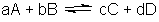The equilibrium constant is equal to the ratio of concentrations, raised to the power of their stiochiometric coefficient, between products and reactants. This value will be constant (for a given fixed temperatue) and can be represented by the following equation: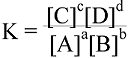So for the previous example of ammonia formation the expression is given as: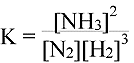So looking at the equation you can see if more ammonia were added to the system the value of the ratio would increase. So to return the value to the original value of K, the system must convert ammonia into nitrogen and hydrogen.